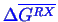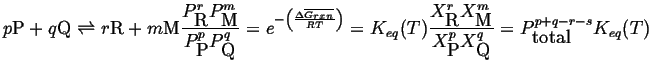Next: Mathematical Thermodynamics Up: Continuum Thermodynamics Previous: Gibbs Free Energy

## Chemical Reaction Equilibria

One immediate consequence of Gibbs free energy being minimized is that we can calculate the equilibrium concentrations in a chemical reaction from the molar change in Gibbs free energy of the reaction. The resulting equation is a fraction containing chemical activities raised to stoichiometric powers is equal to an equilibrium constant that can be calculated from.

This minimization leads directly to what may be a familiar formula for the equilibrium concentrations in a chemical reaction, i.e.,(02-2)

W. Craig Carter 2002-09-05